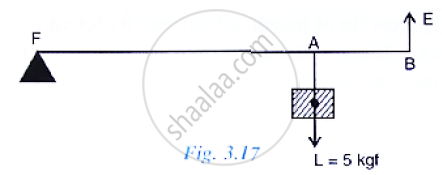Share
Notifications

View all notifications
Books Shortlist
Your shortlist is empty

# Fig 3.17 Below Shows a Lever in Use.(A) to Which Class of Lever Does It Belong? (B) If Fa = 80 Cm, Ab = 20 Cm, Find Its Mechanical Advantage. (C) Calculate the Value of E. - ICSE Class 10 - Physics

Login
Create free account

Forgot password?

#### Question

Fig 3.17 below shows a lever in use.(a) To which class of lever does it belong?
(b) If FA = 80 cm, AB = 20 cm, find its mechanical advantage.
(c) Calculate the value of E.

#### Solution

(a) This is a class II lever.
(b) Given: FA=80 cm, AB = 20 cm, BF= FA+AB=100cm
Mechanical advantage M.A = "𝐵𝐹"/"𝐴𝐹"= 100/80 = 1.25
(c) Effort (E) = "𝐿𝑜𝑎𝑑 (𝐿)"/"𝑀.𝐴" = 5 /1.25 = 4 𝐾𝑔𝑓

Is there an error in this question or solution?

#### APPEARS IN

Solution Fig 3.17 Below Shows a Lever in Use.(A) to Which Class of Lever Does It Belong? (B) If Fa = 80 Cm, Ab = 20 Cm, Find Its Mechanical Advantage. (C) Calculate the Value of E. Concept: Concept of Lever.
S